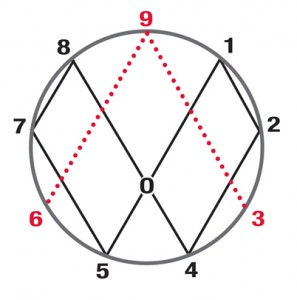top of page
Search

# Star tetrahedron fractal energy(Star tetrahedron)=3:3

3:3=30:30=Dodecahedron

3:3=33=1+1+2+3+5+8+13

3=triangle=(3,6,9)=energy

(Star tetrahedron)=18=6:6:6=3³=27

27=(124875+(369))=(fingerprint of god)=torus

(Star tetrahedron)=(seed of life)

Fingerprint of god:Fingerprint of god enneagram torus:Enneagram=(star tetrahedron)=(tree of life(E8xE8))=tetragrammaton=tetractys=120-cell=(infinite dodecahedron star fractal)

(Infinite dodecahedron star fractal)=infinite(3,6,9)=(infinite energy)

(8 tree of life's)=(64 tetrahedron grid)